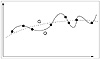# 98.C++ Neural Networks and Fuzzy Logic by Valluru B. Rao M&T Books, IDG Books Worldwide, Inc. ISBN: 1558515526   Pub Date: 06/01/95

Generalization versus Memorization

If your overall goal is beyond pattern classification, you need to track your network’s ability to generalize. Not only should you look at the overall error with the training set that you define, but you should set aside some training examples as part of a test set (and do not train with them), with which you can see whether or not the network is able to correctly predict. If the network responds poorly to your test set, you know that you have overtrained, or you can say the network “memorized” the training patterns. If you look at the arbitrary curve-fitting analogy in Figure 14.2, you see curves for a generalized fit, labeled G, and an overfit, labeled O. In the case of the overfit, any data point outside of the training data results in highly erroneous prediction. Your test data will certainly show you large error in the case of an overfitted model.Figure 14.2  General (G) versus over fitting (0) of data.

Another way to consider this issue is in terms of Degrees Of Freedom (DOF). For the polynomial:

y= a0 + a1x + a2x2 + anxn...

the DOF equals the number of coefficients a0, a1 ... an, which is N + 1. So for the equation of a line (y=a0 + a1x), the DOF would be 2. For a parabola, this would be 3 and so on. The objective to not overfit data can be restated as an objective to obtain the function with the least DOF that fits the data adequately. For neural network models, the larger the number of trainable weights (which is a function of the number of inputs and the architecture), the larger the DOF. Be careful with having too many (unimportant) inputs. You may find terrific results with your training data, but extremely poor results with your test data.

#### Eliminate Correlated Inputs Where Possible

You have seen that getting to the minimum number of inputs for a given problem is important in terms of minimizing DOF and simplifying your model. Another way to reduce dimensionality is to look for correlated inputs and to carefully eliminate redundancy. For example, you may find that the Swiss franc and German mark are highly correlated over a certain time period of interest. You may wish to eliminate one of these inputs to reduce dimensionality. You have to be careful in this process though. You may find that a seemingly redundant piece of information is actually very important. Mark Jurik, of Jurik Consulting, in his paper on data preprocessing, suggests that one of the best ways to determine if an input is really needed is to construct neural network models with and without the input and choose the model with the best error on training and test data. Although very iterative, you can try eliminating as many inputs as possible this way and be assured that you haven’t eliminated a variable that really made a difference.

Another approach is sensitivity analysis, where you vary one input a little, while holding all others constant and note the effect on the output. If the effect is small you eliminate that input. This approach is flawed because in the real world, all the inputs are not constant. Jurik’s approach is more time consuming but will lead to a better model.

The process of decorrelation, or eliminating correlated inputs, can also utilize a linear algebra technique called principal component analysis. The result of principal component analysis is a minimum set of variables that contain the maximum information. For further information on principal component analysis, you should consult a statistics reference or research two methods of principal component analysis: the Karhunen-Loev transform and the Hotelling transform.

#### Design a Network Architecture

Now it’s time to actually design the neural network. For the backpropagation feed-forward neural network we have designed, this means making the following choices:

1.  The number of hidden layers.
2.  The size of hidden layers.
3.  The learning constant, beta([beta]).
4.  The momentum parameter, alpha([alpha]).
5.  The form of the squashing function (does not have to be the sigmoid).
6.  The starting point, that is, initial weight matrix.

Some of the parameters listed can be made to vary with the number of cycles executed, similar to the current implementation of noise. For example, you can start with a learning constant [beta] that is large and reduce this constant as learning progresses. This allows rapid initial learning in the beginning of the process and may speed up the overall simulation time.

#### The Train/Test/Redesign Loop

Much of the process of determining the best parameters for a given application is trial and error. You need to spend a great deal of time evaluating different options to find the best fit for your problem. You may literally create hundreds if not thousands of networks either manually or automatically to search for the best solution. Many commercial neural network programs use genetic algorithms to help to automatically arrive at an optimum network. A genetic algorithm makes up possible solutions to a problem from a set of starting genes. Analogous to biological evolution, the algorithm combines genetic solutions with a predefined set of operators to create new generations of solutions, who survive or perish depending on their ability to solve the problem. The key benefit of genetic algorithms (GA) is the ability to traverse an enormous search space for a possibly optimum solution. You would program a GA to search for the number of hidden layers and other network parameters, and gradually evolve a neural network solution. Some vendors use a GA only to assign a starting set of weights to the network, instead of randomizing the weights to start you off near a good solution.C++ Neural Networks and Fuzzy Logic
ISBN: 1558515526
EAN: 2147483647
Year: 1995
Pages: 139

Similar book on Amazon